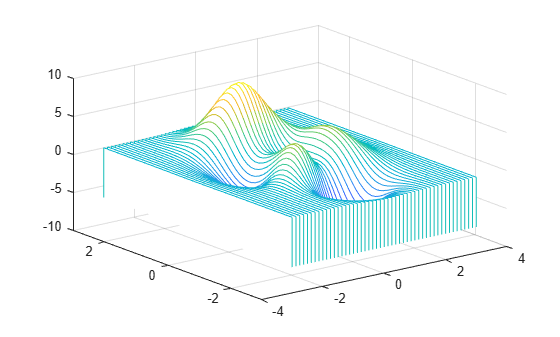# waterfall

Waterfall plot

•## Syntax

``waterfall(X,Y,Z)``
``waterfall(X,Y,Z,C)``
``waterfall(Z)``
``waterfall(Z,C)``
``waterfall(ax,___)``
``p = waterfall(___)``

## Description

example

````waterfall(X,Y,Z)` creates a waterfall plot, which is a mesh plot with a partial curtain along the y dimension. This results in a "waterfall" effect. The function plots the values in matrix `Z` as heights above a grid in the xy-plane defined by `X` and `Y`. The edge colors vary according to the heights specified by `Z`.```

example

````waterfall(X,Y,Z,C)` additionally specifies the color of the edges.```
````waterfall(Z)` creates a waterfall plot, and uses the column and row indices of the elements in `Z` as the x- and y-coordinates.```
````waterfall(Z,C)` additionally specifies the color of the edges.```
````waterfall(ax,___)` plots into the axes specified by `ax` instead of the current axes. Specify the axes as the first input argument. This argument can be used with any of the previous input syntaxes.```

example

````p = waterfall(___)` returns the patch object. Use `p` to modify the waterfall plot after it is created. For a list of properties, see Patch Properties.```

## Examples

collapse all

Create three matrices of the same size. Then plot them as a waterfall plot. The mesh plot uses `Z` for both height and color.

```[X,Y] = meshgrid(-3:.125:3); Z = peaks(X,Y); waterfall(X,Y,Z)```Specify the colors for a waterfall plot by including a fourth matrix input, `C`. The waterfall plot uses `Z` for height and `C` for color. Add a color bar to the graph to show how the data values in `C` correspond to the colors in the colormap.

```[X,Y] = meshgrid(-3:.125:3); Z = peaks(X,Y); C = gradient(Z); waterfall(X,Y,Z,C) colorbar```Create a waterfall plot. To allow further modifications, assign the patch object to the variable `p`.

```[X,Y] = meshgrid(-5:.5:5); Z = Y.*sin(X) - X.*cos(Y); p = waterfall(X,Y,Z)``````p = Patch with properties: FaceColor: [1 1 1] FaceAlpha: 1 EdgeColor: 'flat' LineStyle: '-' Faces: [21x26 double] Vertices: [546x3 double] Show all properties ```

Use `p` to access and modify properties of the waterfall plot after it is created. For example, change the color of the plot edges by setting the `EdgeColor` property.

`p.EdgeColor = 'b';`Display a partial curtain along the x-dimension (instead of the y-dimension) by transposing the input arguments.

```[X,Y] = meshgrid(-3:.125:3); Z = peaks(X,Y); waterfall(X',Y',Z')```## Input Arguments

collapse all

x-coordinates, specified as a matrix the same size as `Z`, or as a vector with length n when `Z` is an m-by-n matrix. If you do not specify values for `X` and `Y`, `waterfall` uses the vectors `(1:n)` and `(1:m)`.

The `XData` property of the patch object stores the x-coordinates.

Example: `X = 1:10`

Example: `X = [1 2 3; 1 2 3; 1 2 3]`

Example: `[X,Y] = meshgrid(-5:0.5:5)`

Data Types: `single` | `double` | `int8` | `int16` | `int32` | `int64` | `uint8` | `uint16` | `uint32` | `uint64` | `categorical`

y-coordinates, specified as a matrix the same size as `Z` or as a vector with length m when `Z` is an m-by-n matrix. If you do not specify values for `X` and `Y`, `waterfall` uses the vectors `(1:n)` and `(1:m)`.

The `YData` property of the patch object stores the y-coordinates.

Example: `Y = 1:10`

Example: `Y = [1 1 1; 2 2 2; 3 3 3]`

Example: `[X,Y] = meshgrid(-5:0.5:5)`

Data Types: `single` | `double` | `int8` | `int16` | `int32` | `int64` | `uint8` | `uint16` | `uint32` | `uint64` | `categorical`

z-coordinates, specified as a matrix. `Z` must have at least two rows and two columns.

`Z` specifies the height of the waterfall plot at each xy-coordinate. If you do not specify the colors, then `Z` also specifies the plot edge colors.

The `ZData` property of the patch object stores the z-coordinates.

Example: `Z = [1 2 3; 4 5 6]`

Example: `Z = sin(x) + cos(y)`

Data Types: `single` | `double` | `int8` | `int16` | `int32` | `int64` | `uint8` | `uint16` | `uint32` | `uint64` | `categorical`

Color indexing array, specified as an m-by-n matrix of colormap indices, where `Z` is m-by-n. For each grid point on the waterfall plot, `C` indicates a color in the colormap. The `CDataMapping` property of the patch object controls how the values in `C` correspond to colors in the colormap.

The `CData` property of the patch object stores the color array. For additional control over the plot coloring, use the `FaceColor` and `EdgeColor` properties.

Target axes, specified as an `Axes` object. If you do not specify the axes, then `waterfall` plots into the current axes.

## Tips

• To analyze the data as columns instead of rows, call `waterfall` with transposed arguments:

```[X,Y] = meshgrid(-3:.125:3); Z = peaks(X,Y); waterfall(X',Y',Z')```

• To create a mesh surface object instead of a patch object, use the `meshz` function. To create a plot similar to a waterfall plot, set the `MeshStyle` property of the surface to `'Row'`.

## Algorithms

The range of `X`, `Y`, and `Z`, or the current setting of the axes' `XLim`, `YLim`, and `ZLim` properties, determines the range of the axes (also set by `axis`). The range of `C`, or the current setting of the axes' `CLim` property, determines the color scaling (also set by `caxis`).

## Version History

Introduced before R2006a